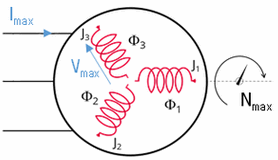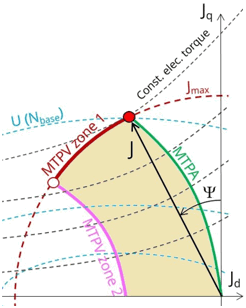# Flux e-Machine Toolbox: Input parameters

## Introduction

After having opened the coupling component in Flux, the user must define several input parameters before running a test. These parameters allow to:

• pilot the solving of the Flux project
• specify solving options (distribution, memory)
• compute and build the different performance maps## Input parameters : Wished data• Maximum phase current RMS
• Maximum phase voltage RMS
• Command mode:
• either MPTA: Maximum Torque Per Ampere with the standard solving workflow
• either MTPV: Maximum Torque Per Volt with the standard solving workflow
• either MTPA Fast: Maximum Torque Per Ampere with the fast solving workflow
• either MTPV Fast: Maximum Torque Per Volt with the fast solving workflow
• Maximum allowed rotating speed for the electrical machine

## Input parameters: Rotor initial angle

The Rotor initial angle is a necessary variable in order to apply Park’s transforms. Two possibilities:

• User: the user knows the initial angle of the rotor and can enter the value
• Auto: the value of the initial angle of the rotor is computed automatically. The goal is to find the rotor angle for t=0s in order to align it with the magnetic flux density in the airgap generated by the reference phase (e.g., phase A). Two simulations are performed in order to compute rotor initial angle. A path in the middle of the airgap is automatically defined in order to compute the magnetic flux density within it.

## Input parameters: Number of computations requested

• With the standard solving workflow - command modes MTPA and MTPV:
• Number of computations for Jd and Jq (8 by default)
• Number of computations for the speed (10 by default)
• Number of computations per electrical period (30 by default)
Note: The total number of computations is the product of the 4, so with the default values there are 19200 computation points. It is necessary to have enough points to obtain a good Torque-Speed envelope. So the calculation time can therefore be quite long. The choice of values is a compromise between accuracy of results and solving time. The default values allow this compromise.
• With the fast solving workflow - command modes MTPA Fast and MTPV Fast:
• Number of computations for Jd and Jq (8 by default)
Note: For these two command modes MTPA Fast and MTPV Fast
• The parametric solving process is done only on the parameters Jd and Jq.
• It is not possible to modify the Number of computations for the speed, nor the Number of computations per electrical period. These two fields are disabled (grayed out).

With this fast solving workflow (MTPA Fast and MTPV Fast command modes), the computation is faster than with the standard solving workflow (MTPA and MTPV command modes).

## Input parameters: Options of distribution and memory

• Activate or not the local distribution (on Windows and on Linux since 2022.1 version).Attention: Make sure that the distribution has been configured. If this is not the case even with the option to Yes, the solving will be sequential and not distributed.

Note: The computing distribution is not implemented in Skew.
• Numerical memory allocated to the solving process of the Flux project:

Since FeMT 2021, there is a new memory mode to run tests: the Dynamic memory mode.

From now on, there are two memory modes: User (static) and Dynamic.

• If the User (static) memory mode is used, then the numerical memory value (in MiB) allocated to the solving process of the Flux project is displayed. The user can modify this value.On the other hand, the user can switch to the Dynamic numerical memory mode by entering 0.Note: For FeMT versions prior to 2022.1, the user could not switch to the Dynamic memory mode in FeMT. In order to run tests in Dynamic memory mode, the user had to regenerate the coupling component from a Flux running in Dynamic memory mode.
• If the Dynamic memory mode is used, then Dynamic is displayed and the numerical memory used is dynamically managed by Flux during its execution. So in this mode, the user does not set any value for the numerical memory.On the other hand, the user can switch to the User numerical memory mode by entering a numerical value in MiB.

Warning: When switching from Dynamic numerical memory mode to User numerical memory mode, the user must know the numerical memory required to solve the project and therefore set a high enough value.Note: For FeMT versions prior to 2022.1, the user could not switch to the User memory mode in FeMT. In order to run tests in User memory mode, the user had to regenerate the coupling component from a Flux running in User memory mode.
• Number of cores used by the Mumps solver for the initial project
• If the local distribution is Yes, then the value of the number of cores used by the Mumps solver is fixed at 1, and non accessible for the user, (in this case the parameter is grayed out, which means that the indicated value is not taken into account)
• If the local distribution is No, then the value of the number of cores entered is taken into account by the Mumps solver. By default this value is 4.
• In the case where the local distribution is Yes, but the distribution has not been correctly configured, then the number of cores entered will be taken into account by the Mumps solver.